# Operators in Python with Examples

Operators in Python work the same as in mathematics, that is, operators such as +, -, *, etc. are used to perform operations. Now these are the two questions that arise in our minds:

1. What operations are going to be performed?
2. Where are the operations performed?

The answers to these two questions are:

1. The operator used determines it.
2. on variables and values.

For example, the following program demonstrates the addition operation (using +) on two variables and the subtraction operation (using -) on two values:

```a = 20
b = 10
c = a + b
print(c)

res = 100 - 50
print(res)```

This program produces the output shown in the snapshot given below: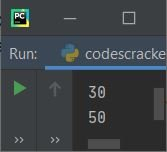As you can see, the addition operation is performed on a and b, and whatever the result is, it gets initialized to the "c" variable. And in the second case, the subtraction operation is performed directly on two values, which are 100 and 50, and again, whatever the result value comes out as, it gets initialized to the "res" variable.

Note: For operator precedence and operator modules, refer to their separate tutorials.

## Types of Operators in Python

Operators that are used in Python are divided into seven categories, as listed here:

Now let's discuss all these operators in brief with well-designed example programs for each category.

## Arithmetic operators in Python

To perform arithmetical operations in a Python program, we have arithmetic operators. The table given below lists all the arithmetic operators available, along with syntax to use and examples. The example is given with the variables' values as a = 5 and b = 3:

Operator Name Syntax Output
+ Addition c = a + b c = 8
- Subtraction c = a - b c = 2
* Multiplication c = a * b c = 15
/ Division c = a / b c = 1.6666666666666667
% Modulus c = a % b c = 2
** Exponentiation c = a ** b c = 125
// Floor Division c = a // b c = 1

Important: The difference between the / (division) and // (floor division) operators is that the division operator divides and returns the exact division result value, where the floor division operator divides and then takes the floor value of the result value. For example, 5/3 gives 1.666666666667, whereas 5//3 gives 1. Here the floor value indicates an integer value (a whole number) that is less than or equal to the original result.

Note: When the ** operator applies to a variable or a value, for example, a ** b, it gets treated as ab. Therefore, 5 ** 3 gets treated as 53, which gives 125 as its result.

The program given below uses all the arithmetic operators. This program is created to clear all your remaining doubts (if any):

```a = 5
b = 3
c = a + b
print(a, "+", b, "=", c)
c = a - b
print(a, "-", b, "=", c)
c = a * b
print(a, "*", b, "=", c)
c = a / b
print(a, "/", b, "=", c)
c = a % b
print(a, "%", b, "=", c)
c = a ** b
print(a, "*", b, "=", c)
c = a // b
print(a, "//", b, "=", c)```

The snapshot given below shows the sample output produced by this program:## Assignment operators in Python

To assign (set) or re-assign the new value to a variable in Python programming, we have another category of operator named the assignment operator. The table given below lists all those operators used for assigning purposes, along with their syntax and examples.

Operator Name Syntax Output
= Simple Assign b = a If a = 5
Then, b = 5
+= Add then Assign b += a If a = 5, b = 0
Then, b = 5
-= Subtract then Assign b -= a If a = 5, b = 12
Then, b = 7
*= Multiply then Assign b *= a If a = 5, b = 2
Then, b = 10
/= Divide then Assign b /= a If a = 5, b = 14
Then, b = 2.8
%= Modulo then Assign b %= a If a = 5, b = 27
Then, b = 2
//= Floor Divide then Assign b //= a If a = 5, b = 14
Then, b = 2
**= Exponent then Assign b **= a If a = 5, b = 2
Then, b = 32
&= Bitwise AND then Assign b &= a If a = 10, b = 4
Then, b = 0
|= Bitwise OR then Assign b |= a If a = 10, b = 4
Then, b = 14
^= Bitwise XOR then Assign b ^= a If a = 10, b = 4
Then, b = 14
>>= Bitwise Right Shift then Assign b >>= a If a = 1, b = 10
Then, b = 5
<<= Bitwise Left Shift then Assign b <<= a If a = 1, b = 10
Then, b = 20

The program given below implements all the above operators. This program was designed to help you better understand assignment operators by demonstrating the following:

```a = 5
b = a
print("Simple Assign Result:", b)

a = 5
b = 0
b += a

a = 5
b = 12
b -= a
print("Subtract then Assign Result:", b)

a = 5
b = 2
b *= a
print("Multiply then Assign Result:", b)

a = 5
b = 14
b /= a
print("Divide then Assign Result:", b)

a = 5
b = 27
b %= a
print("Modulo then Assign Result:", b)

a = 5
b = 14
b //= a
print("Floor Divide then Assign Result:", b)

a = 5
b = 2
b **= a
print("Exponent then Assign Result:", b)

a = 10
b = 4
b &= a
print("Bitwise AND then Assign Result:", b)

a = 10
b = 4
b |= a
print("Bitwise OR then Assign Result:", b)

a = 10
b = 4
b ^= a
print("Bitwise XOR then Assign Result:", b)

a = 1
b = 10
b >>= a
print("Bitwise Right Shift then Assign Result:", b)

a = 1
b = 10
b <<= a
print("Bitwise Left Shift then Assign Result:", b)```

Here is the output that this Python program of assignment operators produced: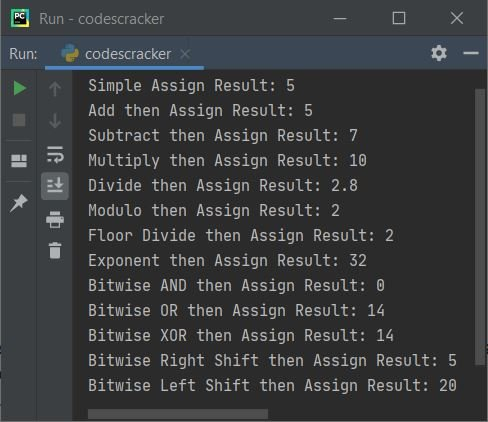In the assignment operator, the expression:

`b += a`

is basically the short version of:

`b = b + a`

## Comparison operators in Python

Comparison operators are used in Python programming to compare two variables or directly values.These operators are used to compare two entities. The table given below shows all the comparison operators along with the syntax to use and an example. The example is taken with two variables, namely, a and b. The values of these two variables are a = 12 and  b = 7.

Operator Name Syntax Output
== Equal a == b False
!= Not equal a != b True
> Greater than a > b True
< Less than a < b False
>= Greater than or equal to a >= b True
<= Less than or equal to a <= b False

The program given below uses all these comparison operators. Since the comparison operators are used to compare two values, therefore, on printing the comparison result, we'll only get the output in the form of "True" or "False." For example, the statement "a < b" returns True if the value of a is less than b, otherwise False gets returned. Let's have a look at the example given below:

```a = 12
b = 7
print("\n---------", a, "==", b, "?--------")
print(a == b)
print("\n---------", a, "!=", b, "?--------")
print(a != b)
print("\n---------", a, ">", b, "?--------")
print(a > b)
print("\n---------", a, "<", b, "?--------")
print(a < b)
print("\n---------", a, ">=", b, "?--------")
print(a >= b)
print("\n---------", a, "<=", b, "?--------")
print(a <= b)```

Here is the output produced: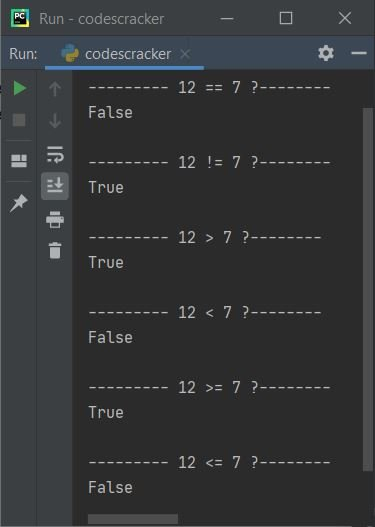## Logical operators in Python

To connect two or more expressions and evaluate their logical output, we have another operator category named the logical operator. The table given below shows all the logical operators along with their syntax and output. The initial values of three variables, namely a, b, and c, are a = 12, b = 7, and c = 9.

Operator Syntax Returns
and a > b and a < c False
or a > b or a < c True
not not(a > b) False

Now the example given below uses all three logical operators:

```a = 12
b = 7
c = 9
print(a > b and a < c)
print(a > b or a < c)
print(not(a > b))```

And here is the output produced:## Bitwise operators in Python

This section includes all the bitwise operators that can be used in the Python language. To learn how all of these Bitwise operators perform operations, see Bitwise Operators with Example, which covers everything about Bitwise operators in a nutshell. For now, the table given below shows all bitwise operators along with syntax and output. The example taken with two variables, namely a and b, whose values are supposed to be a = 10 and b = 3, is

Operator Name Syntax Output
& Bitwise AND a & b 2
| Bitwise OR a | b 11
^ Bitwise XOR a ^ b 9
˜ Bitwise NOT ˜a -11
<< Bitwise Left Shift a << b 1
>> Bitwise Right Shift a >> b 80

The example given below uses all these bitwise operators:

```a = 10
b = 3
print(a, "&", b, "=", a & b)
print(a, "|", b, "=", a | b)
print(a, "^", b, "=", a ^ b)
print("˜", a, "=", ˜a)
print(a, ">>", b, "=", a >> b)
print(a, "<<", b, "=", a << b)```

The output that this program generates is: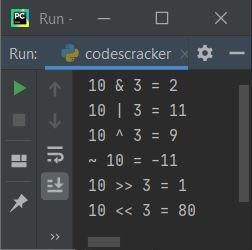## Identity operators in Python

To determine whether a variable or a value is of a certain type or class or not in Python programming, we've got another category of operators called the identity operator. That is basically used to identify variables or values. The table given below lists identity operators that can be used in Python, along with syntax and examples. The example is taken with a and b variables whose values are a=[1, 2, 3], b=[4, 5]:

Operator Syntax Returns
is a is b False
is not a is not b True

The example given below implements these two identity operators available in Python:

```a = [1, 2, 3]
b = [4, 5]
c = a
print(a is b)
print(a is c)
print(a is not b)
print(a is not c)```

The image below displays an example of the output this Python program produced: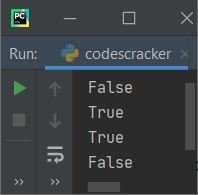## Membership operators in Python

This is the last category of operator used in Python. This category of operators is basically used to check whether a specific value is a member of any specific list, string, or tuple or not. The table given below lists membership operators with syntax, examples, and output. The example taken with a variable named a whose initial value is a = [1, 2, 3] is:

Operator Syntax Example Output
in element in a 2 in a True
not in element not in a 4 in a False

Let's have a look at the example given below to better understand the above two membership operators:

```a = [1, 2, 3]
print(2 in a)
print(4 in a)
print(2 not in a)
print(4 not in a)```

The screenshot provided below displays a sample of the output this program produced: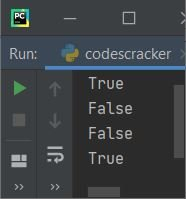Let's have a look at another example that shows the famous use of the membership operator (the "in" operator) in Python programming:

```a = ["python", "operators", "tutorial"]
print("Enter an element to check for existence in list: ")
elem = input()
if elem in a:
print("\nIt is available")
else:
print("\nIt is not available")```

And here is the sample output produced by this program after supplying "Python" as input:#### More Examples

Here is a list of some more examples that use operators in Python:

Python Online Test

« Previous Tutorial Next Tutorial »